Question

In 2005, 1,475,623 students heading to college took the SAT. The distribution of scores in the math section of the SAT follows a normal distribution with mean
µ = 520 and population standard deviation = 115.

What math SAT score is 1.5 standard deviations above the mean? Round answer to a whole number.

1.A math SAT score of 693 is 1.5 standard deviations above the mean

Step-by-step explanation:

Normal Probability Distribution

Problems of normal distributions can be solved using the z-score formula.

In a set with mean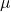and standard deviation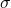, the z-score of a measure X is given by: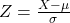The Z-score measures how many standard deviations the measure is from the mean. After finding the Z-score, we look at the z-score table and find the p-value associated with this z-score. This p-value is the probability that the value of the measure is smaller than X, that is, the percentile of X. Subtracting 1 by the p-value, we get the probability that the value of the measure is greater than X.

Mean µ = 520 and population standard deviation = 115.

This means thatWhat math SAT score is 1.5 standard deviations above the mean?

This is X when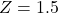. So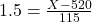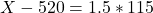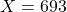A math SAT score of 693 is 1.5 standard deviations above the mean